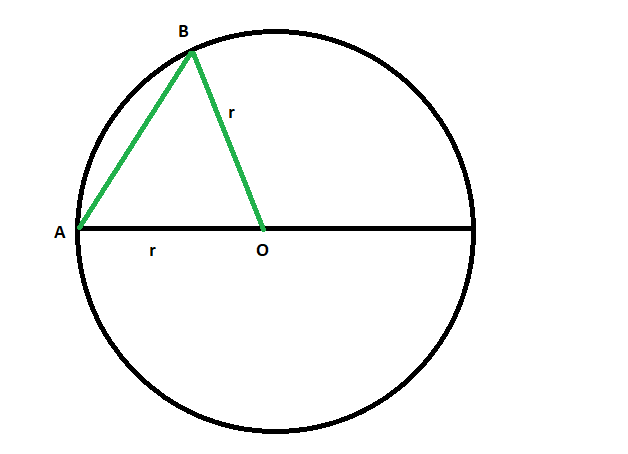Open in App
Not now

# Find the Diameter or Longest chord of a Circle

• Last Updated : 07 Jun, 2022

Given a circle with radius ‘r’ is given, the task is to find the diameter or longest chord of the circle.
Examples:

```Input: r = 4
Output: 8

Input: r = 9
Output: 18```Proof that the Longest chord of a circle is its Diameter:

• Draw circle O and any chord AB on it.
• From one endpoint of the chord, say A, draw a line segment through the centre. That is, draw a diameter.
• Now draw a radius from centre O to B.
• By the triangle inequality,

```AB < AO + OB
= r + r
= 2r
= d```
• So, any chord that is not a diameter will be smaller than a diameter.
• So the largest chord is a diameter

Approach:

• The Longest chord of any circle is its diameter.
• Therefore, the diameter of a circle is twice the radius of it.

`Length of the longest chord or diameter = 2r`
•

Below is the implementation of the above approach:

## C++

 `// C++ program to find``// the longest chord or diameter``// of the circle whose radius is given` `#include ``using` `namespace` `std;` `// Function to find the longest chord``void` `diameter(``double` `r)``{``    ``cout << ``"The length of the longest chord"``         ``<< ``" or diameter of the circle is "``         ``<< 2 * r << endl;``}` `// Driver code``int` `main()``{` `    ``// Get the radius``    ``double` `r = 4;` `    ``// Find the diameter``    ``diameter(r);` `    ``return` `0;``}`

## Java

 `// Java program to find``// the longest chord or diameter``// of the circle whose radius is given``class` `GFG``{``    ` `// Function to find the longest chord``static` `void` `diameter(``double` `r)``{``    ``System.out.println(``"The length of the longest chord"``        ``+ ``" or diameter of the circle is "``        ``+ ``2` `* r);``}` `// Driver code``public` `static` `void` `main(String[] args)``{``    ` `    ``// Get the radius``    ``double` `r = ``4``;` `    ``// Find the diameter``    ``diameter(r);``}``}` `// This code contributed by Rajput-Ji`

## Python3

 `# Python3 program to find``# the longest chord or diameter``# of the circle whose radius is given` `# Function to find the longest chord``def` `diameter(r):` `    ``print``(``"The length of the longest chord"``        ``,``" or diameter of the circle is "``        ``,``2` `*` `r)`  `# Driver code` `# Get the radius``r ``=` `4` `# Find the diameter``diameter(r)` `# This code is contributed by mohit kumar`

## C#

 `// C# program to find``// the longest chord or diameter``// of the circle whose radius is given``using` `System;` `class` `GFG``{``    ` `// Function to find the longest chord``static` `void` `diameter(``double` `r)``{``    ``Console.WriteLine(``"The length of the longest chord"``        ``+ ``" or diameter of the circle is "``        ``+ 2 * r);``}` `// Driver code``public` `static` `void` `Main(String[] args)``{``    ` `    ``// Get the radius``    ``double` `r = 4;` `    ``// Find the diameter``    ``diameter(r);``}``}` `// This code has been contributed by 29AjayKumar`

## PHP

 ``

## Javascript

 ``

Output:

`The length of the longest chord or diameter of the circle is 8`

Time Complexity: O(1)

Auxiliary Space: O(1)

My Personal Notes arrow_drop_up